# Percentages Problems

#### Number of problems found: 684

• Percentage increaseIncrease number 400 by 3.5%
• NumberWhat number is 20 % smaller than the number 198?
• The hockey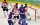The hockey player scored 6 goals from 15 shots. How many % was he successful?
• WalkingOf the 450 students at the school, 432 walks to school. What percentage is this?
• Perctentages35% of what number is 35?
• Vinegar 2How many percentages of vinegar solution will we get if we mix 3.5 liters of 5.8% and 5 liters of 7.6% vinegar?
• Selling price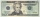Find the selling price. Cost to store: \$50 Markup: 10%
• PercentCalculate how many % is the number 26.25 less than the number 105.
• IronIron ore contains 54% iron. How much ore is needed to produce 23 tons of iron?
• ProbabilityHow probable is a randomly selected three-digit number divisible by five or seven?
• Interest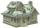What is the annual interest rate on your account if we put 32790 and after 176 days received 33939.2?
• TVsProduction of television sets increased from 3,500 units to 4,200 units. Calculate the percentage of production increase.
• Percents - easyHow many percent is 432 out of 434?
• Profitability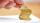The purchase price of goods is 13000, the sales price is the 20000. What is the profitability as a percentage?
• CacaoCacao contains 34% filling. How many grams of filling are in 130 g cacao.
• Base, percents, valueBase is 344084 which is 100 %. How many percent is 384177?
• Find sales tax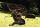What is 8.25% sales tax out of 1607.00?
• Car valueThe car loses value 15% every year. Determine a time (in years) when the price will be halved.
• Bureaucracy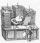The office was attended by 23 people. 9 of them completed the questionnaire. What is the percentage of all people?
• ShotsDetermine the percentage rate of keeper interventions if from 32 shots doesn't caught four shots.

Do you have an interesting mathematical word problem that you can't solve it? Submit a math problem, and we can try to solve it.

We will send a solution to your e-mail address. Solved examples are also published here. Please enter the e-mail correctly and check whether you don't have a full mailbox.

Please do not submit problems from current active competitions such as Mathematical Olympiad, correspondence seminars etc...